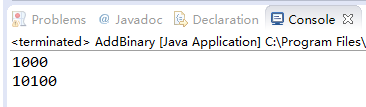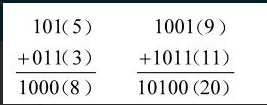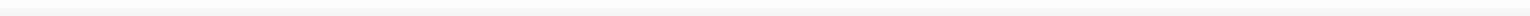• ## 二进制加法运算

千次阅读 2019-06-20 21:54:54
两个二进制整数相加时，是位对位处理的，从最低的一对位（右边）开始，依序将每一对位进行加法运算。两个二进制数字相加，有四种结果，如下所示： 0 + 0 = 0 0 + 1 = 1 1 + 0 = 1 1 + 1 = 10 1 与 1 ...
两个二进制整数相加时，是位对位处理的，从最低的一对位（右边）开始，依序将每一对位进行加法运算。两个二进制数字相加，有四种结果，如下所示：

0 + 0 = 0
0 + 1 = 1
1 + 0 = 1
1 + 1 = 10
1 与 1 相加的结果是二进制的 10（等于十进制的 2）。多出来的数字向更高位产生一个进位。如下图所示，两个二进制数 0000 0100 和 0000 0111 相加：从两个数的最低位（位 0）开始，计算 0+1，得到底行对应位上的 1。然后计算次低位（位 1）。在位 2 上，计算 1+1，结果是 0，并产生一个进位 1。然后计算位 3，0+0，还要加上位 2 的进位，结果是 1。

其余的位都是 0。上图右边是等价的十进制数值加法（4 + 7 = 11），可以用于验证左边的二进制加法。

有些情况下，最高有效位会产生进位。这时，预留存储区的大小就显得很重要。比如，如果计算 1111 1111 加 0000 0001，就会在最高有效位之外产生一个 1，而和数的低 8 位则为全 0。

如果和数的存储大小最少有 9 位，那么就可以将和数表示为 1 0000 0000。但是，如果和数只能保存 8 位，那么它就等于 0000 0000，也就是计算结果的低 8 位。

汇编语言基本概念简介
1.1 汇编语言是什么
1.2 汇编语言的应用
1.3 虚拟机是什么
1.4 汇编语言的数据表示
1.5 二进制整数
1.6 二进制加法运算
1.7 字节（byte）
1.8 十六进制整数
1.9 补码及进制转换
1.10 二进制减法运算

展开全文程序员 编程语言 汇编语言
• 二进制整数的Java实现任意两个二进制数(不论在什么位置)相加，只可能出现4种情况。它们是：0+0=01+0=0+1=11+1=10=0+向高一位的进位11+1+1=11=1+向高一位的进位1//整数二进制相加public static String add(String b1,...
二进制整数的Java实现任意两个二进制数(不论在什么位置)相加，只可能出现4种情况。它们是：0+0=01+0=0+1=11+1=10=0+向高一位的进位11+1+1=11=1+向高一位的进位1//整数二进制相加public static String add(String b1, String b2) {int len1 = b1.length();int len2 = b2.length();String s1 = b1;String s2 = b2;StringBuffer sb1 = new StringBuffer();//先将位数较少的二进制高位补零if(len1 > len2) {for(int i = 0; i < (len1 - len2); i++) {sb1.append(0);}sb1.append(b2);s1 = b1;s2 = sb1.toString();} else if(len1 < len2) {for(int j = 0; j < (len2 - len1); j++) {sb1.append(0);}sb1.append(b1);s1 = sb1.toString();s2 = b2;}//进位int flag = 0;StringBuffer sb2 = new StringBuffer();for(int z = s1.length() - 1; z >= 0; z--) {//字符’0’的对应的ASCII十进制是48//分情况判断if((s1.charAt(z) - 48) == 0 && (s2.charAt(z) - 48) == 0) {sb2.append(flag);flag = 0;continue;}if(((s1.charAt(z) - 48) == 0 && (s2.charAt(z) - 48) == 1 && flag == 0) || ((s1.charAt(z) - 48) == 1 && (s2.charAt(z) - 48) == 0 && flag == 0)) {sb2.append(1);flag = 0;continue;}if(((s1.charAt(z) - 48) == 0 && (s2.charAt(z) - 48) == 1 && flag == 1) || ((s1.charAt(z) - 48) == 1 && (s2.charAt(z) - 48) == 0 && flag == 1)) {sb2.append(0);flag = 1;continue;}if((s1.charAt(z) - 48) == 1 && (s2.charAt(z) - 48) == 1 && flag == 0) {sb2.append(0);flag = 1;continue;}if((s1.charAt(z) - 48) == 1 && (s2.charAt(z) - 48) == 1 && flag == 1) {sb2.append(1);flag = 1;}}if(flag == 1) {sb2.append(flag);}//倒置sb2.reverse();return sb2.toString();}测试public static void main(String[] args) {System.out.println(add("101", "011"));System.out.println(add("1001", "1011"));}展开全文• 先看打印结果在看代码比较好理解。结果在最下面的位置。总结：讲解了二进制的按位异域、按位与、左移的运算规则。并通过次3种算法得到2个数...//初始化要执行加法的值System.out.println("===开始二进制运算===");a...
先看打印结果在看代码比较好理解。结果在最下面的位置。总结：讲解了二进制的按位异域、按位与、左移的运算规则。并通过次3种算法得到2个数相加的结果。二进制应该还有其他算法，由于知识浅薄就不知道了。代码：public static void main(String[] args){int a=11,b=9;//初始化要执行加法的值System.out.println("===开始二进制运算===");add(a,b);System.out.println("===二进制运算结束===");}/*** 将二进制的数据打印出来* @param binars 需要打印的二进制值* @param paramName 需要打印的参数名称* @return 返回打印的字符串*/public  static String printBnary(StringBuilder binars,int param){StringBuilder binarsRe=new StringBuilder();String[] strs=binars.toString().split(",");int strLength=strs.length;while (strLength>=1){binarsRe.append(strs[strLength-1]+" ");strLength--;}return binarsRe.toString();}/*** 十进制转换为二进制输出* @param a 要转换的十进制数* @param binars 接收转换后的二进制数据* @param paramName 需要打印的参数名称* @return*/public static  String DecimalTransBinary(int a,StringBuilder binars,int param){int rem=a%2;//取余数int base=a/2;//取基数/*** 物流是余数和基数都必须除尽为0*/if(base>0 || rem>0){//将所有的余数都添加到字符串中使用，隔开在打印是使用binars.append(rem+",");DecimalTransBinary(base,binars,param);}//十进制转换二进制成功后，将二进制数据打印出来return printBnary(binars,param);}/*** 十进制加法，解释如何使用二进制实现的* @param a 执行加法的参数* @param b 执行加法的参数* @return 返回加法成功后的整数*/public static  int add(int a,int b){//对需要相加的数据输出为二进制StringBuilder binars=new StringBuilder();String aStr=DecimalTransBinary(a,binars,a);//将stringBuilder数据输出到控制台binars.setLength(0);String bStr=DecimalTransBinary(b,binars,b);int res=a;//按为异域的二进制运算int xor=a^b;System.out.println("");System.out.println("按位异域(^)：二进制数据的相同位不同则为1，相同则为0。");System.out.println(a+"的二进制值："+aStr);System.out.println(b+"的二进制值："+bStr);System.out.println("参数："+a+" 参数："+b+"  进行"+a+"^"+b+":");binars.setLength(0);String xorStr=DecimalTransBinary(xor,binars,xor);System.out.println(aStr+"^"+bStr+"="+xorStr);System.out.println("按位异域后得到的二进制值："+xorStr);System.out.println("按位异域后得到的十进制值："+xor);//按位与的二进制运算和左移一位的值int forward=(a&b)<<1;System.out.println();System.out.println("按位与(&)：二进制数据的相同位都为1则为1，若有一个不为1则为0。");System.out.println("左移(<System.out.println("10110转换为十进制就是：22。左移用十进制解释就是，11乘以2的1次方的结果。11<<2就是11乘以2的次方的结果。");System.out.println("参数："+a+" 参数："+b+"  进行("+a+"&"+b+")<<1:");binars.setLength(0);String forwardStr=DecimalTransBinary(forward,binars,forward);System.out.println("("+aStr+"&"+bStr+")<<1="+forwardStr);System.out.println("按位与后得到的二进制值："+forwardStr);System.out.println("按位与后得到的十进制值："+forward);System.out.println();if(forward!=0){System.out.print("("+a+"&"+b+")<<1="+forward+"运算结果不为0，");System.out.println("将"+a+"^"+b+"运算的结果值："+xor+";("+a+"&"+b+")<<1运算的结果值："+forward+"。继续重复以上运算。");res=add(xor,forward);}else{System.out.println("("+a+"&"+b+")<<1="+forward+"运算结果为0；"+a+"^"+b+"按位异域的运算结果就是最终的和："+xor);res=xor;}return  res;}结果：===开始二进制运算===按位异域(^)：二进制数据的相同位不同则为1，相同则为0。11的二进制值：1 0 1 19的二进制值：1 0 0 1参数：11 参数：9进行11^9:1 0 1 1 ^1 0 0 1 =1 0按位异域后得到的二进制值：1 0按位异域后得到的十进制值：2按位与(&)：二进制数据的相同位都为1则为1，若有一个不为1则为0。左移(<10110转换为十进制就是：22。左移用十进制解释就是，11乘以2的1次方的结果。11<<2就是11乘以2的次方的结果。参数：11 参数：9进行(11&9)<<1:(1 0 1 1 &1 0 0 1 )<<1=1 0 0 1 0按位与后得到的二进制值：1 0 0 1 0按位与后得到的十进制值：18(11&9)<<1=18运算结果不为0，将11^9运算的结果值：2;(11&9)<<1运算的结果值：18。继续重复以上运算。按位异域(^)：二进制数据的相同位不同则为1，相同则为0。2的二进制值：1 018的二进制值：1 0 0 1 0参数：2 参数：18进行2^18:1 0 ^1 0 0 1 0 =1 0 0 0 0按位异域后得到的二进制值：1 0 0 0 0按位异域后得到的十进制值：16按位与(&)：二进制数据的相同位都为1则为1，若有一个不为1则为0。左移(<10110转换为十进制就是：22。左移用十进制解释就是，11乘以2的1次方的结果。11<<2就是11乘以2的次方的结果。参数：2 参数：18进行(2&18)<<1:(1 0 &1 0 0 1 0 )<<1=1 0 0按位与后得到的二进制值：1 0 0按位与后得到的十进制值：4(2&18)<<1=4运算结果不为0，将2^18运算的结果值：16;(2&18)<<1运算的结果值：4。继续重复以上运算。按位异域(^)：二进制数据的相同位不同则为1，相同则为0。16的二进制值：1 0 0 0 04的二进制值：1 0 0参数：16 参数：4进行16^4:1 0 0 0 0 ^1 0 0 =1 0 1 0 0按位异域后得到的二进制值：1 0 1 0 0按位异域后得到的十进制值：20按位与(&)：二进制数据的相同位都为1则为1，若有一个不为1则为0。左移(<10110转换为十进制就是：22。左移用十进制解释就是，11乘以2的1次方的结果。11<<2就是11乘以2的次方的结果。参数：16 参数：4进行(16&4)<<1:(1 0 0 0 0 &1 0 0 )<<1=按位与后得到的二进制值：按位与后得到的十进制值：0(16&4)<<1=0运算结果为0；16^4按位异域的运算结果就是最终的和：20===二进制运算结束===
展开全文• 展开全部我有点忙，所以没时间给你慢慢改。不过我这儿有一个计算器的代码，你不介意的话用吧！import java.awt.*;import java.awt.event.*;import javax.swing.*;public class Calculator implements ActionListener...
展开全部我有点忙，所以没时间给你慢慢改。不过我这儿有一个计算器的代码，你不介意的话用吧！import java.awt.*;import java.awt.event.*;import javax.swing.*;public class Calculator implements ActionListener{private double data1=0.0,data2=0.0;private String t_content;boolean number=false;//key用来标示用62616964757a686964616fe59b9ee7ad9431333264656665户所按下的按钮即加减乘除//key为0标示+，为1表示-，为2表示*，为3表示/short key=-1;//pkey的取值为-1~5//pkey为-1表示第一次按下=按钮，为5说明不是第一次按下=按钮//pkey为其他值0,1,2,3时分别代表+，-,*，/short pkey=-1;Frame frame=new Frame("Calculator");//定义标题为Calculator的窗体TextField textfield=new TextField(30);//定义计算机//定义backspace,ce,c0按钮Button backspace=new Button("Backspace");Button ce=new Button("CE");Button c0=new Button("C");//定义面板，其中backspace,ce,c0按钮和计算机兰textfeild放在面板p1中//其余按钮放在面板p2中Panel p1=new Panel();Panel p2=new Panel();//定义界面上的按钮数组，即除去backspace,ce,c0按钮的所有按钮String names[]=;Button bb[]=new Button[names.length];public static void main(String[] args){Calculator cal=new Calculator();cal.go();}public void go(){frame.setSize(300,200);frame.setLayout(new BorderLayout());Font fonts=new Font("楷体_GB2312",Font.PLAIN,12);//设置面板p1的字体和布局管理类型，将textfeild对象添加到面板中p1.setFont(fonts);p1.setLayout(new GridLayout(2,1,5,10));textfield.setFont(fonts);p1.add(textfield,null);//backspace,ce,c0按钮注册addActionListener方法backspace.addActionListener(this);ce.addActionListener(this);c0.addActionListener(this);//将backspace,ce,c0按钮添加到p1面板中p1.add(backspace,null);p1.add(ce,null);p1.add(c0,null);//设置面板p2的布局管理器类型为GridLayoutp2.setLayout(new GridLayout(4,5,5,5));//为按钮组中的按钮注册addActionListener方法for(int i=0;ibb[i]=new Button(names[i]);p2.add(bb[i]);bb[i].addActionListener(this);}//设置面板p1和面板p2在窗体中的位置//面板p1在窗体的North方向，面板p2在窗体的South方向frame.add(p1,BorderLayout.NORTH);frame.add(p2,BorderLayout.SOUTH);frame.setVisible(true);//当单击关闭按钮时退出系统frame.addWindowListener(new WindowAdapter(){public void windowClosing(WindowEvent e){System.exit(0);}});}public void actionPerformed(ActionEvent ae){String s;//通过ActionEvent类的getActionCommand()方法，得出动作是由哪个按钮触发的。                                                                    s=ae.getActionCommand();//用户单击一次，计算栏里便去掉一个字符//先将计算栏里的字符清空，再添加除最好一个字符的字符串if(s.equals("Backspace")&&number){t_content=textfield.getText();textfield.setText("");for(int i=0;ichar c=t_content.charAt(i);textfield.setText(textfield.getText()+c);}}//用户单击的是CE按钮，计算栏清空if(s.equals("CE")){textfield.setText("");number=false;}//用户单击的是C按钮if(s.equals("C")){textfield.setText("");data1=data2=0.0;number=false;}//用户单击的是sqrt按钮，其中负数不能被开方if(s.equals("sqrt")&&number){t_content=textfield.getText();if(t_content.charAt(0)=='-'){textfield.setText("负数不能被开方");number=false;}else{textfield.setText(Double.toString(java.lang.Math.sqrt(Double.parseDouble(t_content))));}}//用户单击的是1/x求倒数按钮，求倒数时分母不能为0if(s.equals("1/x") && number){t_content=textfield.getText();if(t_content.equals("0")){                                                      textfield.setText("零不能求倒数");number=false;}else{textfield.setText(Double.toString(1/Double.parseDouble(t_content)));}}//用户单击的是%按钮if(s.equals("%")&&number){if(data2==0){t_content=textfield.getText();textfield.setText(t_content);}else{boolean g=false;for(int i=0;iif('.'==textfield.getText().charAt(i)){g=true;break;}//如果是Double 数，除100if(g=true){double dtemp=Double.parseDouble(textfield.getText());dtemp=dtemp/100.0;textfield.setText(Double.toString(dtemp));}//如果是int数但能被100整除，则去掉末尾两个0else{if(Integer.parseInt(textfield.getText())%100==0){int itemp=Integer.parseInt(textfield.getText());itemp/=100;textfield.setText(Integer.toString(itemp));}//如果是int数，但不能被100整除，则按Double数处理else{double dtemp=Double.parseDouble(textfield.getText());dtemp=dtemp/100.0;textfield.setText(Double.toString(dtemp));}}}                                                                    }//对正负数字的处理if(s.equals("+/-")){t_content=textfield.getText();if(t_content.charAt(0)=='-'){textfield.setText("");String content;for(int i=1;itextfield.setText(textfield.getText()+t_content.charAt(i));}}else{textfield.setText("-"+t_content);}}//所按下的按钮为+按钮if(s.equals("+")){switch(pkey){case 0:data2+=Double.parseDouble(textfield.getText());break;case 1:data2+=Double.parseDouble(textfield.getText());break;case  2:data2+=Double.parseDouble(textfield.getText());break;case 3:if(textfield.getText().equals("0")){textfield.setText("除数不能为零");number=false;}else{key=-1;data2/=data1;textfield.setText(Double.toString(data2));break;}default:data2=Double.parseDouble(textfield.getText());}pkey=key=0;textfield.setText("");}//所按下的按钮为-按钮if(s.equals("-")){switch(pkey){case 0:data2+=Double.parseDouble(textfield.getText());break;case 1:data2+=Double.parseDouble(textfield.getText());break;case  2:data2+=Double.parseDouble(textfield.getText());break;case 3:if(textfield.getText().equals("0")){textfield.setText("除数不能为零");number=false;}else{key=-1;data2/=data1;textfield.setText(Double.toString(data2));break;}default:data2=Double.parseDouble(textfield.getText());}pkey=key=1;textfield.setText("");}//所按下的按钮为*按钮if(s.equals("*")){switch(pkey){case 0:data2+=Double.parseDouble(textfield.getText());break;case 1:data2+=Double.parseDouble(textfield.getText());break;case  2:data2+=Double.parseDouble(textfield.getText());break;case 3:if(textfield.getText().equals("0")){                                                         textfield.setText("除数不能为零");number=false;}else{key=-1;data2/=data1;textfield.setText(Double.toString(data2));break;}default:data2=Double.parseDouble(textfield.getText());}pkey=key=2;textfield.setText("");}//所按下的按钮为/按钮if(s.equals("/")){switch(pkey){case 0:data2+=Double.parseDouble(textfield.getText());break;case 1:data2+=Double.parseDouble(textfield.getText());break;case  2:data2+=Double.parseDouble(textfield.getText());break;case 3:if(textfield.getText().equals("0")){textfield.setText("除数不能为零");number=false;}else{key=-1;data2/=data1;textfield.setText(Double.toString(data2));break;}default:data2=Double.parseDouble(textfield.getText());}pkey=key=3;textfield.setText("");}//所按下的按钮为=，求结果if(s.equals("=")&&number){//如果不是第一次按下=按钮if(pkey==5){switch(pkey){case 0:data2+=data1;textfield.setText(Double.toString(data2));break;case 1:data2-=data1;textfield.setText(Double.toString(data2));break;case 2:data2*=data1;textfield.setText(Double.toString(data2));break;case 3:if(textfield.getText().equals("0")){textfield.setText("除数不能为零");number=false;}else{data2/=data1;textfield.setText(Double.toString(data2));break;}}}//如果是第一次按下=按钮else{data1=Double.parseDouble(textfield.getText());switch(key){case 0:key=-1;data2+=Double.parseDouble(textfield.getText());textfield.setText(Double.toString(data2));break;case 1:key=-1;data2-=Double.parseDouble(textfield.getText());textfield.setText(Double.toString(data2));                                     break;case 2:key=-1;data2*=Double.parseDouble(textfield.getText());textfield.setText(Double.toString(data2));break;case 3:if(textfield.getText().equals("0")){textfield.setText("除数不能为零");number=false;}else{key=-1;data2/=Double.parseDouble(textfield.getText());textfield.setText(Double.toString(data2));break;}}}pkey=5;}//按下的按钮时数字按钮else{switch(s.charAt(0)){case'0':case'1':case'2':case'3':case'4':case'5':case'6':case'7':case'8':case'9':number=true;textfield.setText(textfield.getText()+s);break;//对.的处理case'.':t_content=textfield.getText();boolean g=false;                                                    //对计算机栏中的数据进行检验//如果其中有小数点，则用户单击该按钮时不在添加小数点for(int i=0;iif(t_content.charAt(i)=='.'){g=true;break;}}//数据中已经有小数点if(g==true)break;// 数据中没有小数点，当用户单击小数点时添加小数点if(g==false)textfield.setText(t_content+".");}}}private String Integer(String string) {// TODO Auto-generated method stubreturn null;}}已赞过已踩过<你对这个回答的评价是？评论收起
展开全文• php实现二进制加法： 思路：没有工作中应用过此场景，但十进制的加法还是经常做的，能不能用十进制加法变相实现呢？ 答案是可以的，并且php也提供进制间转换的函数，我的实现使用了 bindec():二进制转十进制；...
• 二进制的算术运算：1、二进制加法：0+0=0，0+1=1，1+0=1，1+1=10(向高位进位)。两个相加的二进制位仅一位为1时，相加的结果为1；如果两个二进制位全是0，相加的结果仍为0；而如果两个相加的二进制位均为1，则结果...
• 题目：两个二进制数a和b，用string字符串表示，要求进行加法运算 解法： string addBinary(string a, string b) { string ret; ret.reserve(a.size()+b.size());//类似于分配内存空间 int c=0,s; int i=a....
• 计算机能够识别的数制是二进制数，二进制数是用0和1两个数字来表示的数，计算机的指令集也是采用二进制表示，机器语言就是用二进制数编写程序。可以借助十进制数来理解二进制数。在十进制中，每一位有0、1、2、3、4...
• 解题思路： a ^ b可以得到两数相加不进位的加法结果 (a & b) << 1可以得到两数相加产生的进位 二进制的加法无外乎就以下几种情况， ...再分析二进制加法中进位怎么能保存，因为只有 1+1的时.c++
• int2binary = {} #用于将输入的整数转为计算机可运行的二进制数用 binary_dim = 8 #定义了二进制数的长度=8 largest_number = pow(2,binary_dim) #二进制数最大能取的数就=256喽 binary = np.unpackbits( np.array...RNN
• 主要内容进制及其转换标识符、关键字常量...人类使用二进制：计算机使用八进制和十六进制：为了更好的表示二进制(缩短二进制长度)各进制数之间的关系1.2 计算机为什么使用二进制物理上容易实现，可靠性强；运算简单...
• 实验一 多位十六进制加法运算实验 张云帆 17308226 电子专业 一. 实验要求 利用Proteus平台，建立8086的多位十六进制加法运算的例子。 . 实验目的 熟悉实验系统的编程和使用。 掌握使用加法类运算指令编程...微机原理实验
• 标签：这两天看了《编码的奥秘》里面的二进制加法机及其后面的减法功能的实现，就用Python实现了一个类似功能的加法器出来。先说一下整体的思想。由于操作数都是二进制，所以计算简单了许多。首先，运算需要逐位操作...
• ## 二进制加法

千次阅读 2007-01-21 18:10:00
题目： 输入两个正整数Ｘ，Ｙ，将Ｘ，Ｙ化为二进制数，然后将这两个二进制数作二进制加法运算，再将结化为十进制数输出。 （二进制加法规则：0+0=0 0+1=1 1+0=1 1+1=10 ）提示：在数组里运算。思路：先将输入的...iostream 语言 c
• 在计算机中只有加法，可以说实现了加法，我们就可以实现减法、乘法、除法甚至更复杂的操作，但是这一切的前提是让机器实现二进制加法运算。二进制的加法其实最简单，但是计算机如何能够实现二进制的加法呢？ 现在...
• 各位值相加，不算进位值，二进制亦或运算 计算进位值，二进制运算，然后左移一位； 对1,2步的结果，重复以上两步骤 两数之和就是不计算进位值加上进位值，直到没有进位 第二部为什么要左移？ 当你在十进制下...
• 二进制加法与十进制加法是类似的，只是规则是“逢二进一”。如十进制的3+5=8，用二进制表示就是11+101=1000.可能还是有一些不懂，我们再来看个例子： 11011+1100=？ 只要记住“逢二进一”，就很清楚结果是100111....计算机基础
• ... 刚刚开始用的转换方法，例子都过了但是...然后用了高精度加法AC，但是注意输出的时候前导0和结果为0的情况 01000001 Time Limit:1000MS Memory Limit:65536K Description Adding bi...
• 这一节主要介绍二进制加法、二进制减法、二进制乘法、二进制除法以及二进制反码、补码等知识。一 二进制加减乘除前面二进制的加减乘除这部分比较基础，也很直接，我就直接复制粘贴老师的PPT了。二 原码、反码和补码...
• ## 二进制加法器

千次阅读 2019-03-17 16:59:52
上一节我们学习了ALU的加法实现功能部件——全加器，进行两个4bit的二进制数相加，就要用到4个全加器（如图1所示）。那么在进行加法运算时，首先准备好的是1号全加器的3个input。而2、3、4号全加器...
•java LeetCode
• 首先我们在做二进制加法运算的时候要清楚，二进制是满2进位，与十进制的满10进位很类似，下面我们来一步步分析下面的图 我们分析的顺序是从右自左，从上自下 1+1=2满2进1下面取0，将进位的1分配给0+1的式子中算法 c语言
• 思路：不用四则运算的话 就想到了用二进制。自己试着实现的时候，断档了。现在来看看学习到的思路：在我们列式计算的时候，各位依次相加，有进位的就进位，其实他是可以分开的，即a+b=（a和b各位相加的值）+（a和b...java...